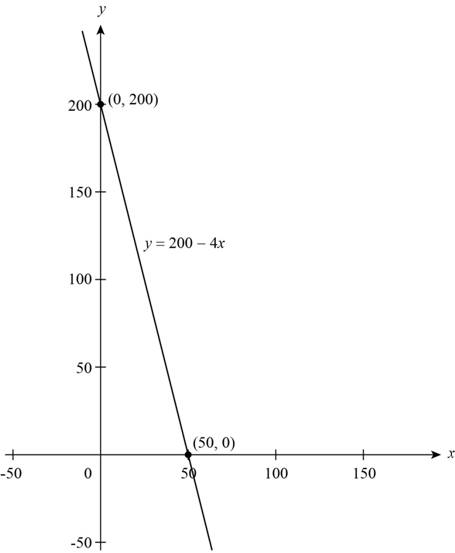# the graph of the given linear equation.### Precalculus: Mathematics for Calcu...

6th Edition
Stewart + 5 others
Publisher: Cengage Learning
ISBN: 9780840068071### Precalculus: Mathematics for Calcu...

6th Edition
Stewart + 5 others
Publisher: Cengage Learning
ISBN: 9780840068071

#### Solutions

Chapter 1.10, Problem 68E

(a)

To determine

## To sketch: the graph of the given linear equation.

Expert Solution

### Explanation of Solution

The linear equation is given,

y=2004x (1)

Where,

y is the number of spaces that manager can rent.

x is the charges for a rental space in dollars.

Calculate x-intercept and y-intercept of line to find the two points of the linear equation that represents a line,

Substitute 0 for y in equation (1) to find the value of x-intercept,

0=2004x4x=200x=2004=50

The first point on the linear equation is (50,0) .

Substitute 0 for x in equation (1) to find the value of y-intercept,

y=20040y=2000=200

The second point on the linear equation is (0,200) .

The equation (1) is in the form of general equation y=mx+b , so the value of slope m is 4 .

Sketch the graph by the points (50,0) , (0,200) and the slope m is 4 .Figure (1)

The graph of linear equation (1) is shown in above Figure (1).

(b)

To determine

Expert Solution

### Explanation of Solution

From part (a), the slope of the linear equation is 4 and the x- intercept is 50 and the y-intercept is 200.

The slope represents a decrease of 4 spaces rented for each one dollar increase in rental price, the y-intercept indicates that 200 spaces are rented if there is no increase in price, and the x-intercept indicates that no space are rented with an increase of \$50 in rental price.

### Have a homework question?

Subscribe to bartleby learn! Ask subject matter experts 30 homework questions each month. Plus, you’ll have access to millions of step-by-step textbook answers!